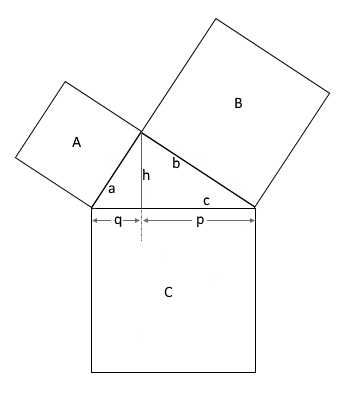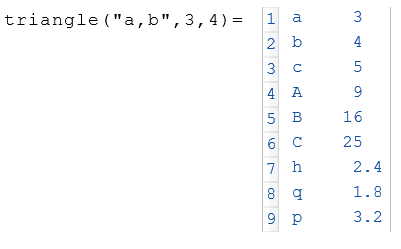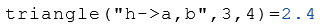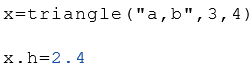# ClassC Triangle

Calculation of the properties of right-angled triangles

## Description

The class Triangle calculates properties of a right triangle.

## Properties

The following properties can be queried as a result or passed as an argument.

#### Description

a Leg ab Leg b
c Hypotenuse
A Area a2
B Area b2
C Area c2
q Hypotenuse sector
p Hypotenuse sector
h Height

## Syntax

Object = Triangle ("arg names", arguments)

Value = Triangle ("get -> arg names", arguments)

## Examples

Calculation of the properties of the side lengths $$a$$ and $$b$$.Calculation of the height $$h$$ from the side lengths $$a$$ and $$b$$.The following example displays the height $$h$$ from the object $$x$$.